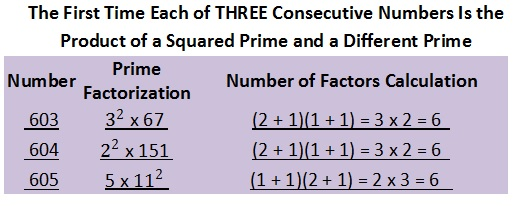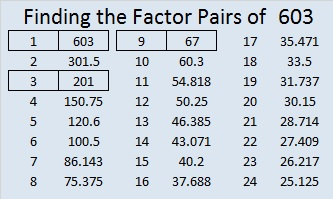# 603 Some Consecutive Numbers and Level 2

603 has six factors. So do the next two counting numbers. Many numbers have exactly six factors, but something unique happens this time.

The most common time a number will have six factors is when that number is the product of a squared prime number and a different prime number. That happens a lot, but this is the first time it has happened to three consecutive numbers!It is NOT the first time that three or even four consecutive numbers had 6 factors because there is also another, less common way for a number to do that: when a number is equal to a prime number raised to the 5th power. Look at this list of consecutive numbers with 6 factors.Between 245 and 603 there are not any other lists of three or more consecutive numbers with six factors.Print the puzzles or type the solution on this excel file: 10 Factors 2015-09-01

—————————————————————————————————

• 603 is a composite number.
• Prime factorization: 603 = 3 x 3 x 67, which can be written 603 = (3^2) x 67
• The exponents in the prime factorization are 2 and 1. Adding one to each and multiplying we get (2 + 1)(1 + 1) = 3 x 2  = 6. Therefore 603 has exactly 6 factors.
• Factors of 603: 1, 3, 9, 67, 201, 603
• Factor pairs: 603 = 1 x 603, 3 x 201, or 9 x 67
• Taking the factor pair with the largest square number factor, we get √603 = (√9)(√67) = 3√67 ≈ 24.556058—————————————————————————————————